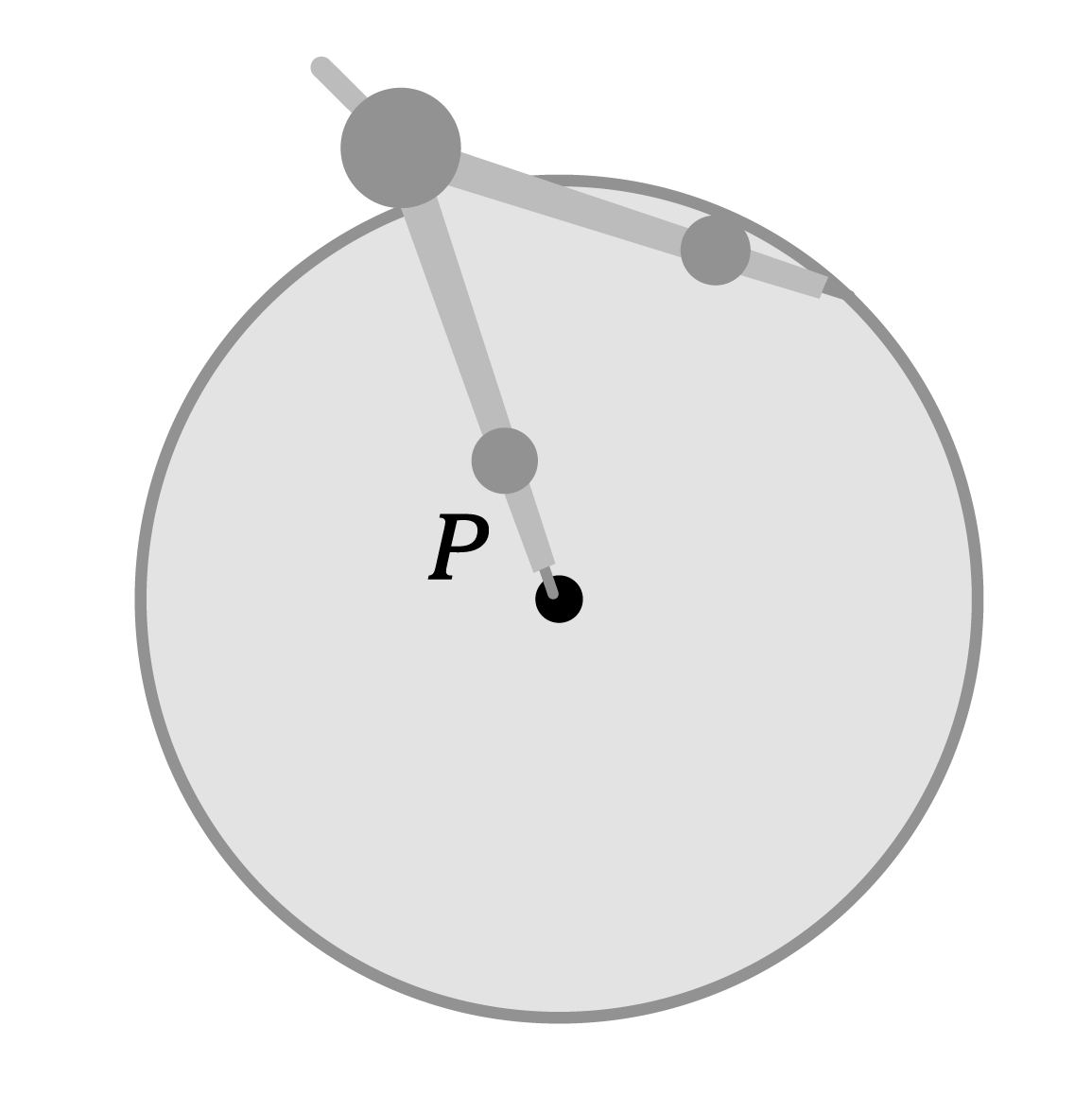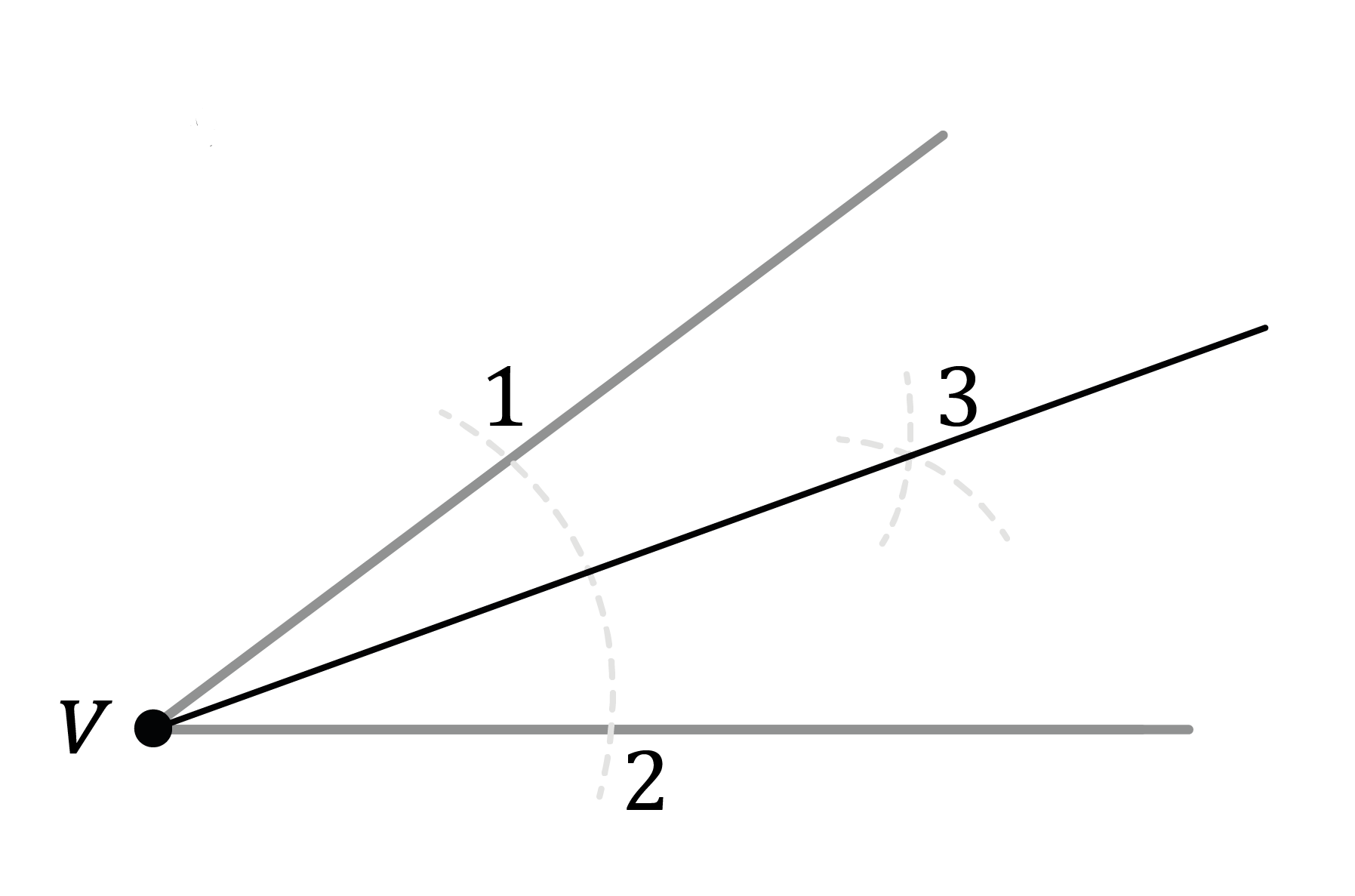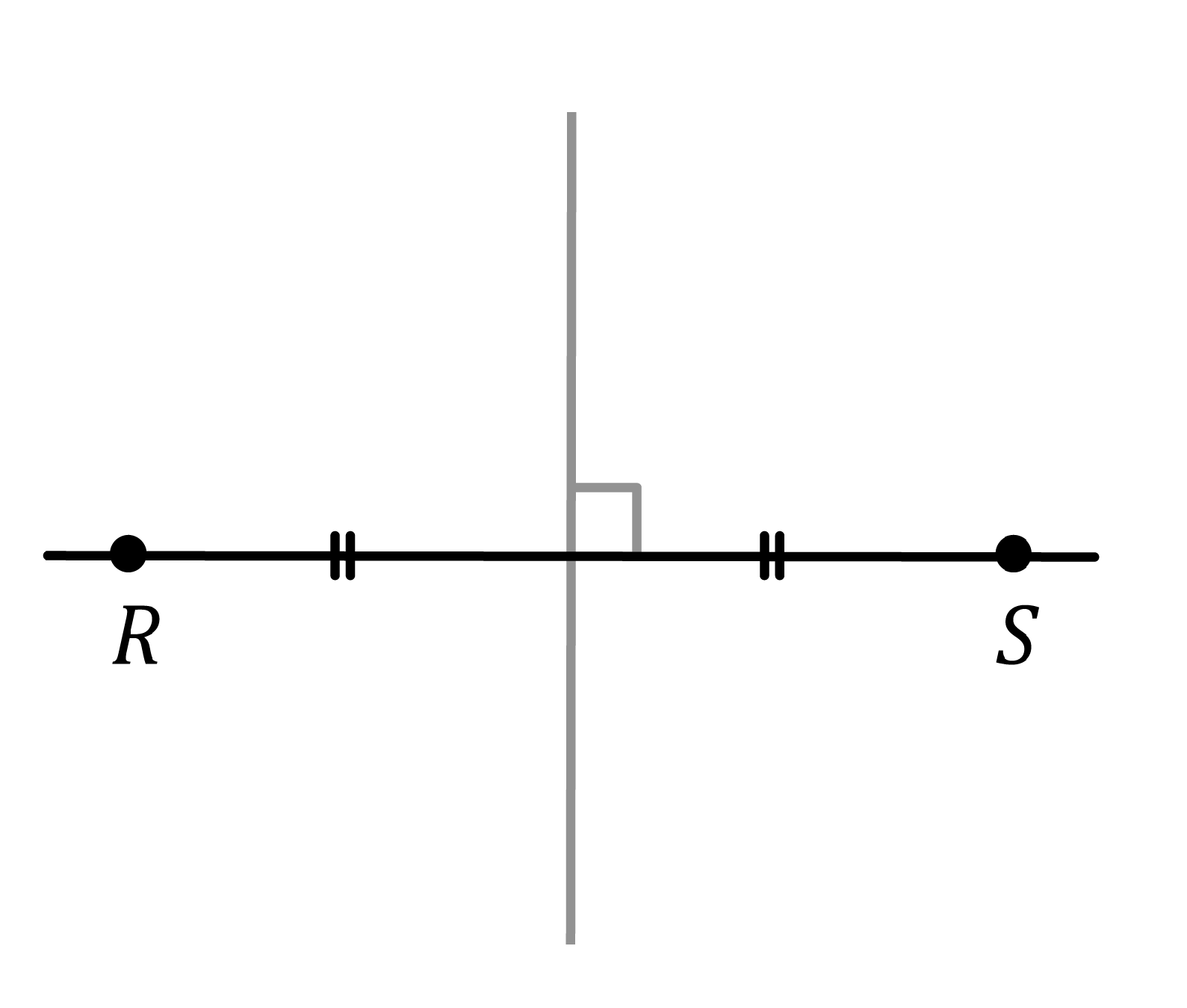Chapter overviewMaths

Exam board

AQA

Number

Algebra

Graphs

Ratio proportion and rates of change

Shapes and area

Angles and geometry

Trigonometry

Probability

Statistics

Maths

# Construction: Loci0%

Summary

# Construction: Loci

## ​​​​In a nutshell

Loci is the plural of locus. A locus is a set of points that obey a certain rule. There are four main loci that you need to be able to perform using a ruler and compass. These four loci can be applied to solve problems in context.

## The four loci

Here are the four loci that you need to know.

 LOCI​ DESCRIPTION DIAGRAM A fixed distance from a given point A circle with the point as the centreA fixed distance from a given line Two semicircles (one around either end of the line) and straight lines connecting the semicirclesEquidistant from two given lines The angle bisector of the two linesEquidistant from two given points The perpendicular bisector of the line that connects the two points### Constructing a fixed distance from a given point

#### ​​procedure

 1 Use the compass and ruler to measure the given distance. 2 Draw a circle of the given distance about the point.

Visually:

### Constructing a fixed distance from a given line

#### ​​procedure

 1 Use the compass and ruler to measure the given distance. 2 Draw circles of the given distance about each end of the line. 3 Draw lines that are parallel to the given line that touch the circles.

Visually:

Note: The procedures for constructing perpendicular and angle bisectors are covered in the lesson Constructions.

## Loci in context

You may have to apply loci to more contextual problems. This may also involve identifying and shading regions.

##### Example

​​In the map below, one box represents one square metre. Shade in the region that is less than $3m$ from the point $A$ and is closer to the point $A$ than point $B$.​

Deal with one locus at a time.

First, identify the locus that is associated with the phrase "less than $3m$".

"Less than $3m$" refers to a circle with radius $3m$.

Construct the relevant locus and mark dashes that indicate the region. In this case, the region is inside the circle.

Do the same with the phrase "closer to point $A$ than point $B$".

"Closer to point $A$ than point $B$" refers to the perpendicular bisector of the line $AB$.

Shade in the region that satisfies all the loci.

## Want to find out more? Check out these other lessons!

Construction: angle and perpendicular bisectors

FAQs

• Question: What are loci?

Answer: Loci is plural for locus. A locus is a set of points that obey a certain rule.

• Question: How many loci are there?

Answer: There are four main loci that you need to know.

• Question: How do you solve contextual loci problems?

Answer: Relate each restriction/rule to a loci and construct all of the loci, marking the region that satisfies all of them.

Theory

Exercises